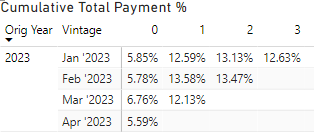cancel
Showing results for
Did you mean:New Member

## How to calculate a correct cumulative percentage

Hi,

In my raw data (group by Year, Vintage and Age), I have two columns called 'total cash' and 'total purchase balance' and I have a measure called 'total payment %' = sum(total cash)/sum(total purchase balance). Below is an example to show the "total payment %"

 Orig Year Vintage age0 age1 age2 age3 2023 Jan, 2023 5.85% 18.21% 14.12% 11.22% Feb, 2023 5.78% 19.65% 13.27% Mar, 2023 6.76% 17.05%

for the next step, I want to calcualte cumulative total payment % so that the results look like below table

 Orig Year Vintage 0 1 2 3 2023 Jan, 2023 5.85% 5.85%+18.21%=24.06% 24.06%+14.12%=38.18% 38.18%+11.22%=49.4% Feb, 2023 5.78% 5.78%+19.65%=25.43% 25.43%+13.27%=38.7% Mar, 2023 6.76% 6.76%+17.05%=23.81%

However, when I use quick measure 'running total' to calculate 'total payment%' over age, I got wrong number:It seems like the measure calculates number based on running sum 'total cash' and sum 'total purchase balance' first and then make the division rather than calculate percentage first and then running sum the percentage:

for example:

if total payment % (Age1) = 10/100=10%, total payment % (age2) = 12/120=10%

my expected cumulative total payment % for age 2 shoud be 10%+10% = 20%

but Power BI return me (10+12)/(100+120)=10%.

Any Idea will be really helpful! thanks a lot

1 ACCEPTED SOLUTIONSuper User

@zhengho , Assume you already have a measure total payment %

Cumm Sales = CALCULATE(Sumx(Values('Date'[Month Year]), [total payment %]),filter(all('Date'),'Date'[date] <=max('Date'[date])))

or

Cumm = CALCULATE(Sumx(values('Date'[Month Year]),[total payment %]) ,filter(allselected('Date'),'Date'[date] <=max('Date'[date])))

2 REPLIES 2Super User

@zhengho , Assume you already have a measure total payment %

Cumm Sales = CALCULATE(Sumx(Values('Date'[Month Year]), [total payment %]),filter(all('Date'),'Date'[date] <=max('Date'[date])))

or

Cumm = CALCULATE(Sumx(values('Date'[Month Year]),[total payment %]) ,filter(allselected('Date'),'Date'[date] <=max('Date'[date])))New MemberAnnouncements#### Exclusive opportunity for Women!

Join us for a free, hands-on Microsoft workshop led by women trainers for women where you will learn how to build a Dashboard in a Day!#### Power Platform Conference-Power BI and Fabric Sessions

Join us Oct 1 - 6 in Las Vegas for the Microsoft Power Platform Conference.Top Solution Authors
Top Kudoed Authors
Users online (1,852)